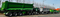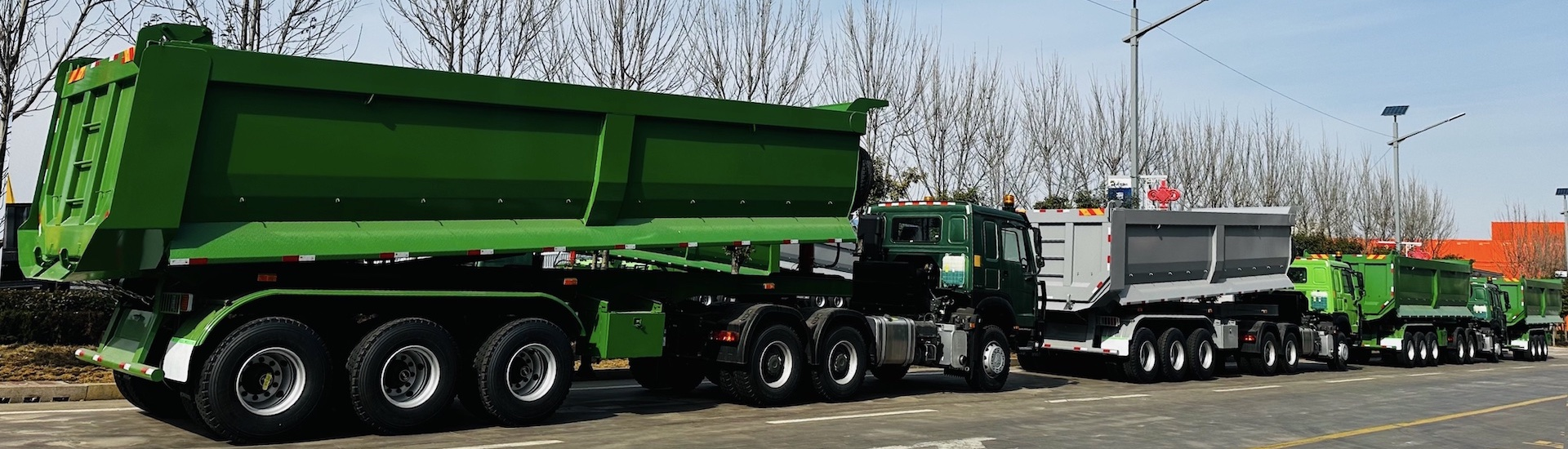English» » How to calculate turning radius of a trailer?

# How to calculate turning radius of a trailer?

Views: 0     Author: Site Editor     Publish Time: 2022-12-16      Origin: SiteMany trailer dealers and distributors often ask how to calculate the turning radius of a trailer. Therefore, we consulted the trailer design experts in our factory to know the calculation method of the trailer turning radius.

When the main vehicle and the trailer form the train to make a turn, in order to make all the wheels roll purely, the axes of all the wheels must intersect at one point, which is called the instantaneous rolling center. The steering center of a wheel-steering three-axle vehicle always moves backward relative to the center of the double rear axle, and the amount of rearward movement, in the formula, l is the center distance between the middle and rear axles, and L is the center distance from the front axle to the middle and rear axles.

l=1100 L=3740

then the amount of backward shift1. Trailer chassis calculation:

Attachment 1

Drawbar rotation angle θ=38°

Kingpin center distance EF=1678

Wheelbase HG=3800

Front wheel base l (wheel)=1800

DH=1050

Substitute into the calculation:

OG=DG ctgθ=(1050+3800) ctg38°=6207

α=arctg[3800÷(6207+1678÷2)]=28.33°

β=arctg[3800÷(6207-1678÷2)]=35.29°

OF=3800÷sinα=8107

R'=OF+(l-EF)÷2=8107+(1800-1727)÷2=8143.5

1. Main car calculation:

Corresponding to the turning radius that the main vehicle EQ2082E6D should achieve:

Tail suspension CK=895 L=3740 a=81

Front wheelbase l (main) = 1774 l (middle and rear wheelbase) = 1100

Substitute into the calculation:

OD=(HG+DH)÷sinθ=(3850+1050)÷sin38°=7959

OC2=OD2+CD2=79592+15302

OC=8104

OB2=OC2-CB2=81042-(895+1100÷2-81)2

OB=7988

R(main)2≈ON2≈［OB+l(wheel)÷2］2+［L+a］2

=[7988+1774÷2]2+[3740+81]2

R(main) ≈9662mm＞8200

The turning radius of the trailer chassis does not match the turning radius of the main vehicle EQ2082E6D, and the turning radius of the main vehicle needs to be adjusted to match.

The above is the calculation method of the trailer radius.